# MATLAB Simulink - Create Subsystem

#### Data Preprocessing for Machine Learning using MATLAB

30 Lectures 4 hours

#### Image Processing Toolbox in MATLAB

17 Lectures 3 hours

#### Matlab - The Complete Course

Best Seller

37 Lectures 5 hours

Subsystems are useful when your model gets big and complex. You can change a part of the model into a subsystem that helps to keep the flow very clean and understandable.

In this chapter, let us learn how to create a simple subsystem in Simulink. First, create a blank model, as shown below −Now, we will create a simple model that adds two numbers and later converts a part of the model into a subsystem.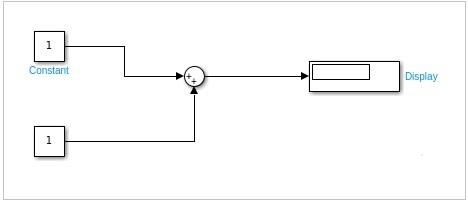We have created a simple model that has two inputs. These inputs will add up and show the result inside the display.

We will change the value of the constant as 10 and 20. The result 10+20 = 30 should be seen inside the display block.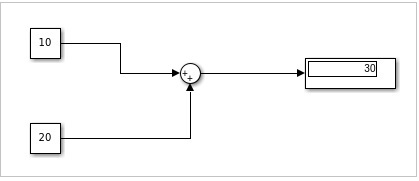Let us add one more block named Unary minus that will change the output from 30 to -30 as shown below −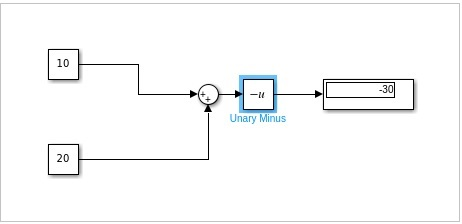Now, let us select the portion i.e. the sum and the Unary minus block to create a subsystem as shown below −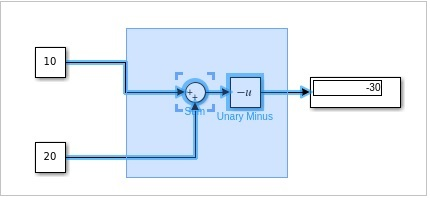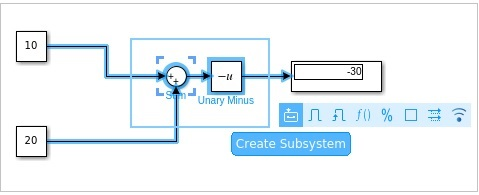Click on Create Subsystem. Once done, the sum block and the Unary minus block will be converted into a subsystem as shown below −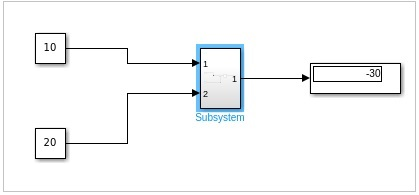Now when you run the simulation, it will display the same result as earlier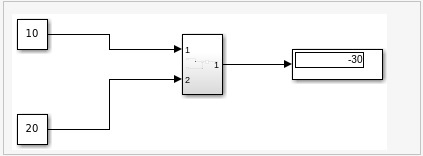Double click on the subsystem to see the original block as shown below −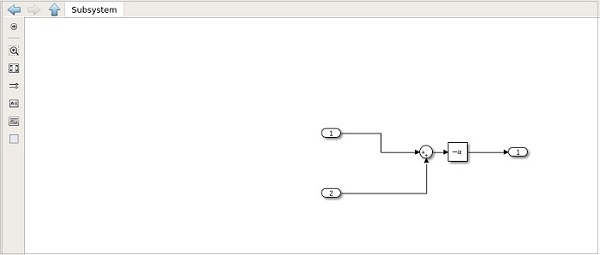Click on the upward arrow close to the subsystem to get back to the model.